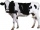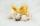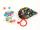# Basket of fruit

In six baskets, the seller has fruit. In individual baskets, there are only apples or just pears with the following number of fruits: 5,6,12,14,23 and 29. "If I sell this basket," the salesman thinks, "then I will have just as many apples as a pear." Which basket did the salesman think?

Result

x =  29

#### Solution:

```j5j6j12j14j23j29 :::H=0; J=89
**** K=5 ** H=0; J=84
**** K=6 ** H=0; J=83
**** K=12 ** H=0; J=77
**** K=14 ** H=0; J=75
**** K=23 ** H=0; J=66
**** K=29 ** H=0; J=60
h5j6j12j14j23j29 :::H=5; J=84
**** K=5 ** H=0; J=84
**** K=6 ** H=5; J=78
**** K=12 ** H=5; J=72
**** K=14 ** H=5; J=70
**** K=23 ** H=5; J=61
**** K=29 ** H=5; J=55
j5h6j12j14j23j29 :::H=6; J=83
**** K=5 ** H=6; J=78
**** K=6 ** H=0; J=83
**** K=12 ** H=6; J=71
**** K=14 ** H=6; J=69
**** K=23 ** H=6; J=60
**** K=29 ** H=6; J=54
h5h6j12j14j23j29 :::H=11; J=78
**** K=5 ** H=6; J=78
**** K=6 ** H=5; J=78
**** K=12 ** H=11; J=66
**** K=14 ** H=11; J=64
**** K=23 ** H=11; J=55
**** K=29 ** H=11; J=49
j5j6h12j14j23j29 :::H=12; J=77
**** K=5 ** H=12; J=72
**** K=6 ** H=12; J=71
**** K=12 ** H=0; J=77
**** K=14 ** H=12; J=63
**** K=23 ** H=12; J=54
**** K=29 ** H=12; J=48
h5j6h12j14j23j29 :::H=17; J=72
**** K=5 ** H=12; J=72
**** K=6 ** H=17; J=66
**** K=12 ** H=5; J=72
**** K=14 ** H=17; J=58
**** K=23 ** H=17; J=49
**** K=29 ** H=17; J=43
j5h6h12j14j23j29 :::H=18; J=71
**** K=5 ** H=18; J=66
**** K=6 ** H=12; J=71
**** K=12 ** H=6; J=71
**** K=14 ** H=18; J=57
**** K=23 ** H=18; J=48
**** K=29 ** H=18; J=42
h5h6h12j14j23j29 :::H=23; J=66
**** K=5 ** H=18; J=66
**** K=6 ** H=17; J=66
**** K=12 ** H=11; J=66
**** K=14 ** H=23; J=52
**** K=23 ** H=23; J=43
**** K=29 ** H=23; J=37
j5j6j12h14j23j29 :::H=14; J=75
**** K=5 ** H=14; J=70
**** K=6 ** H=14; J=69
**** K=12 ** H=14; J=63
**** K=14 ** H=0; J=75
**** K=23 ** H=14; J=52
**** K=29 ** H=14; J=46
h5j6j12h14j23j29 :::H=19; J=70
**** K=5 ** H=14; J=70
**** K=6 ** H=19; J=64
**** K=12 ** H=19; J=58
**** K=14 ** H=5; J=70
**** K=23 ** H=19; J=47
**** K=29 ** H=19; J=41
j5h6j12h14j23j29 :::H=20; J=69
**** K=5 ** H=20; J=64
**** K=6 ** H=14; J=69
**** K=12 ** H=20; J=57
**** K=14 ** H=6; J=69
**** K=23 ** H=20; J=46
**** K=29 ** H=20; J=40 OK OK OK
h5h6j12h14j23j29 :::H=25; J=64
**** K=5 ** H=20; J=64
**** K=6 ** H=19; J=64
**** K=12 ** H=25; J=52
**** K=14 ** H=11; J=64
**** K=23 ** H=25; J=41
**** K=29 ** H=25; J=35
j5j6h12h14j23j29 :::H=26; J=63
**** K=5 ** H=26; J=58
**** K=6 ** H=26; J=57
**** K=12 ** H=14; J=63
**** K=14 ** H=12; J=63
**** K=23 ** H=26; J=40
**** K=29 ** H=26; J=34
h5j6h12h14j23j29 :::H=31; J=58
**** K=5 ** H=26; J=58
**** K=6 ** H=31; J=52
**** K=12 ** H=19; J=58
**** K=14 ** H=17; J=58
**** K=23 ** H=31; J=35
**** K=29 ** H=31; J=29
j5h6h12h14j23j29 :::H=32; J=57
**** K=5 ** H=32; J=52
**** K=6 ** H=26; J=57
**** K=12 ** H=20; J=57
**** K=14 ** H=18; J=57
**** K=23 ** H=32; J=34
**** K=29 ** H=32; J=28
h5h6h12h14j23j29 :::H=37; J=52
**** K=5 ** H=32; J=52
**** K=6 ** H=31; J=52
**** K=12 ** H=25; J=52
**** K=14 ** H=23; J=52
**** K=23 ** H=37; J=29
**** K=29 ** H=37; J=23
j5j6j12j14h23j29 :::H=23; J=66
**** K=5 ** H=23; J=61
**** K=6 ** H=23; J=60
**** K=12 ** H=23; J=54
**** K=14 ** H=23; J=52
**** K=23 ** H=0; J=66
**** K=29 ** H=23; J=37
h5j6j12j14h23j29 :::H=28; J=61
**** K=5 ** H=23; J=61
**** K=6 ** H=28; J=55
**** K=12 ** H=28; J=49
**** K=14 ** H=28; J=47
**** K=23 ** H=5; J=61
**** K=29 ** H=28; J=32
j5h6j12j14h23j29 :::H=29; J=60
**** K=5 ** H=29; J=55
**** K=6 ** H=23; J=60
**** K=12 ** H=29; J=48
**** K=14 ** H=29; J=46
**** K=23 ** H=6; J=60
**** K=29 ** H=29; J=31
h5h6j12j14h23j29 :::H=34; J=55
**** K=5 ** H=29; J=55
**** K=6 ** H=28; J=55
**** K=12 ** H=34; J=43
**** K=14 ** H=34; J=41
**** K=23 ** H=11; J=55
**** K=29 ** H=34; J=26
j5j6h12j14h23j29 :::H=35; J=54
**** K=5 ** H=35; J=49
**** K=6 ** H=35; J=48
**** K=12 ** H=23; J=54
**** K=14 ** H=35; J=40
**** K=23 ** H=12; J=54
**** K=29 ** H=35; J=25
h5j6h12j14h23j29 :::H=40; J=49
**** K=5 ** H=35; J=49
**** K=6 ** H=40; J=43
**** K=12 ** H=28; J=49
**** K=14 ** H=40; J=35
**** K=23 ** H=17; J=49
**** K=29 ** H=40; J=20
j5h6h12j14h23j29 :::H=41; J=48
**** K=5 ** H=41; J=43
**** K=6 ** H=35; J=48
**** K=12 ** H=29; J=48
**** K=14 ** H=41; J=34
**** K=23 ** H=18; J=48
**** K=29 ** H=41; J=19
h5h6h12j14h23j29 :::H=46; J=43
**** K=5 ** H=41; J=43
**** K=6 ** H=40; J=43
**** K=12 ** H=34; J=43
**** K=14 ** H=46; J=29
**** K=23 ** H=23; J=43
**** K=29 ** H=46; J=14
j5j6j12h14h23j29 :::H=37; J=52
**** K=5 ** H=37; J=47
**** K=6 ** H=37; J=46
**** K=12 ** H=37; J=40
**** K=14 ** H=23; J=52
**** K=23 ** H=14; J=52
**** K=29 ** H=37; J=23
h5j6j12h14h23j29 :::H=42; J=47
**** K=5 ** H=37; J=47
**** K=6 ** H=42; J=41
**** K=12 ** H=42; J=35
**** K=14 ** H=28; J=47
**** K=23 ** H=19; J=47
**** K=29 ** H=42; J=18
j5h6j12h14h23j29 :::H=43; J=46
**** K=5 ** H=43; J=41
**** K=6 ** H=37; J=46
**** K=12 ** H=43; J=34
**** K=14 ** H=29; J=46
**** K=23 ** H=20; J=46
**** K=29 ** H=43; J=17
h5h6j12h14h23j29 :::H=48; J=41
**** K=5 ** H=43; J=41
**** K=6 ** H=42; J=41
**** K=12 ** H=48; J=29
**** K=14 ** H=34; J=41
**** K=23 ** H=25; J=41
**** K=29 ** H=48; J=12
j5j6h12h14h23j29 :::H=49; J=40
**** K=5 ** H=49; J=35
**** K=6 ** H=49; J=34
**** K=12 ** H=37; J=40
**** K=14 ** H=35; J=40
**** K=23 ** H=26; J=40
**** K=29 ** H=49; J=11
h5j6h12h14h23j29 :::H=54; J=35
**** K=5 ** H=49; J=35
**** K=6 ** H=54; J=29
**** K=12 ** H=42; J=35
**** K=14 ** H=40; J=35
**** K=23 ** H=31; J=35
**** K=29 ** H=54; J=6
j5h6h12h14h23j29 :::H=55; J=34
**** K=5 ** H=55; J=29
**** K=6 ** H=49; J=34
**** K=12 ** H=43; J=34
**** K=14 ** H=41; J=34
**** K=23 ** H=32; J=34
**** K=29 ** H=55; J=5
h5h6h12h14h23j29 :::H=60; J=29
**** K=5 ** H=55; J=29
**** K=6 ** H=54; J=29
**** K=12 ** H=48; J=29
**** K=14 ** H=46; J=29
**** K=23 ** H=37; J=29
**** K=29 ** H=60; J=0
j5j6j12j14j23h29 :::H=29; J=60
**** K=5 ** H=29; J=55
**** K=6 ** H=29; J=54
**** K=12 ** H=29; J=48
**** K=14 ** H=29; J=46
**** K=23 ** H=29; J=37
**** K=29 ** H=0; J=60
h5j6j12j14j23h29 :::H=34; J=55
**** K=5 ** H=29; J=55
**** K=6 ** H=34; J=49
**** K=12 ** H=34; J=43
**** K=14 ** H=34; J=41
**** K=23 ** H=34; J=32
**** K=29 ** H=5; J=55
j5h6j12j14j23h29 :::H=35; J=54
**** K=5 ** H=35; J=49
**** K=6 ** H=29; J=54
**** K=12 ** H=35; J=42
**** K=14 ** H=35; J=40
**** K=23 ** H=35; J=31
**** K=29 ** H=6; J=54
h5h6j12j14j23h29 :::H=40; J=49
**** K=5 ** H=35; J=49
**** K=6 ** H=34; J=49
**** K=12 ** H=40; J=37
**** K=14 ** H=40; J=35
**** K=23 ** H=40; J=26
**** K=29 ** H=11; J=49
j5j6h12j14j23h29 :::H=41; J=48
**** K=5 ** H=41; J=43
**** K=6 ** H=41; J=42
**** K=12 ** H=29; J=48
**** K=14 ** H=41; J=34
**** K=23 ** H=41; J=25
**** K=29 ** H=12; J=48
h5j6h12j14j23h29 :::H=46; J=43
**** K=5 ** H=41; J=43
**** K=6 ** H=46; J=37
**** K=12 ** H=34; J=43
**** K=14 ** H=46; J=29
**** K=23 ** H=46; J=20
**** K=29 ** H=17; J=43
j5h6h12j14j23h29 :::H=47; J=42
**** K=5 ** H=47; J=37
**** K=6 ** H=41; J=42
**** K=12 ** H=35; J=42
**** K=14 ** H=47; J=28
**** K=23 ** H=47; J=19
**** K=29 ** H=18; J=42
h5h6h12j14j23h29 :::H=52; J=37
**** K=5 ** H=47; J=37
**** K=6 ** H=46; J=37
**** K=12 ** H=40; J=37
**** K=14 ** H=52; J=23
**** K=23 ** H=52; J=14
**** K=29 ** H=23; J=37
j5j6j12h14j23h29 :::H=43; J=46
**** K=5 ** H=43; J=41
**** K=6 ** H=43; J=40
**** K=12 ** H=43; J=34
**** K=14 ** H=29; J=46
**** K=23 ** H=43; J=23
**** K=29 ** H=14; J=46
h5j6j12h14j23h29 :::H=48; J=41
**** K=5 ** H=43; J=41
**** K=6 ** H=48; J=35
**** K=12 ** H=48; J=29
**** K=14 ** H=34; J=41
**** K=23 ** H=48; J=18
**** K=29 ** H=19; J=41
j5h6j12h14j23h29 :::H=49; J=40
**** K=5 ** H=49; J=35
**** K=6 ** H=43; J=40
**** K=12 ** H=49; J=28
**** K=14 ** H=35; J=40
**** K=23 ** H=49; J=17
**** K=29 ** H=20; J=40 OK OK OK
h5h6j12h14j23h29 :::H=54; J=35
**** K=5 ** H=49; J=35
**** K=6 ** H=48; J=35
**** K=12 ** H=54; J=23
**** K=14 ** H=40; J=35
**** K=23 ** H=54; J=12
**** K=29 ** H=25; J=35
j5j6h12h14j23h29 :::H=55; J=34
**** K=5 ** H=55; J=29
**** K=6 ** H=55; J=28
**** K=12 ** H=43; J=34
**** K=14 ** H=41; J=34
**** K=23 ** H=55; J=11
**** K=29 ** H=26; J=34
h5j6h12h14j23h29 :::H=60; J=29
**** K=5 ** H=55; J=29
**** K=6 ** H=60; J=23
**** K=12 ** H=48; J=29
**** K=14 ** H=46; J=29
**** K=23 ** H=60; J=6
**** K=29 ** H=31; J=29
j5h6h12h14j23h29 :::H=61; J=28
**** K=5 ** H=61; J=23
**** K=6 ** H=55; J=28
**** K=12 ** H=49; J=28
**** K=14 ** H=47; J=28
**** K=23 ** H=61; J=5
**** K=29 ** H=32; J=28
h5h6h12h14j23h29 :::H=66; J=23
**** K=5 ** H=61; J=23
**** K=6 ** H=60; J=23
**** K=12 ** H=54; J=23
**** K=14 ** H=52; J=23
**** K=23 ** H=66; J=0
**** K=29 ** H=37; J=23
j5j6j12j14h23h29 :::H=52; J=37
**** K=5 ** H=52; J=32
**** K=6 ** H=52; J=31
**** K=12 ** H=52; J=25
**** K=14 ** H=52; J=23
**** K=23 ** H=29; J=37
**** K=29 ** H=23; J=37
h5j6j12j14h23h29 :::H=57; J=32
**** K=5 ** H=52; J=32
**** K=6 ** H=57; J=26
**** K=12 ** H=57; J=20
**** K=14 ** H=57; J=18
**** K=23 ** H=34; J=32
**** K=29 ** H=28; J=32
j5h6j12j14h23h29 :::H=58; J=31
**** K=5 ** H=58; J=26
**** K=6 ** H=52; J=31
**** K=12 ** H=58; J=19
**** K=14 ** H=58; J=17
**** K=23 ** H=35; J=31
**** K=29 ** H=29; J=31
h5h6j12j14h23h29 :::H=63; J=26
**** K=5 ** H=58; J=26
**** K=6 ** H=57; J=26
**** K=12 ** H=63; J=14
**** K=14 ** H=63; J=12
**** K=23 ** H=40; J=26
**** K=29 ** H=34; J=26
j5j6h12j14h23h29 :::H=64; J=25
**** K=5 ** H=64; J=20
**** K=6 ** H=64; J=19
**** K=12 ** H=52; J=25
**** K=14 ** H=64; J=11
**** K=23 ** H=41; J=25
**** K=29 ** H=35; J=25
h5j6h12j14h23h29 :::H=69; J=20
**** K=5 ** H=64; J=20
**** K=6 ** H=69; J=14
**** K=12 ** H=57; J=20
**** K=14 ** H=69; J=6
**** K=23 ** H=46; J=20
**** K=29 ** H=40; J=20
j5h6h12j14h23h29 :::H=70; J=19
**** K=5 ** H=70; J=14
**** K=6 ** H=64; J=19
**** K=12 ** H=58; J=19
**** K=14 ** H=70; J=5
**** K=23 ** H=47; J=19
**** K=29 ** H=41; J=19
h5h6h12j14h23h29 :::H=75; J=14
**** K=5 ** H=70; J=14
**** K=6 ** H=69; J=14
**** K=12 ** H=63; J=14
**** K=14 ** H=75; J=0
**** K=23 ** H=52; J=14
**** K=29 ** H=46; J=14
j5j6j12h14h23h29 :::H=66; J=23
**** K=5 ** H=66; J=18
**** K=6 ** H=66; J=17
**** K=12 ** H=66; J=11
**** K=14 ** H=52; J=23
**** K=23 ** H=43; J=23
**** K=29 ** H=37; J=23
h5j6j12h14h23h29 :::H=71; J=18
**** K=5 ** H=66; J=18
**** K=6 ** H=71; J=12
**** K=12 ** H=71; J=6
**** K=14 ** H=57; J=18
**** K=23 ** H=48; J=18
**** K=29 ** H=42; J=18
j5h6j12h14h23h29 :::H=72; J=17
**** K=5 ** H=72; J=12
**** K=6 ** H=66; J=17
**** K=12 ** H=72; J=5
**** K=14 ** H=58; J=17
**** K=23 ** H=49; J=17
**** K=29 ** H=43; J=17
h5h6j12h14h23h29 :::H=77; J=12
**** K=5 ** H=72; J=12
**** K=6 ** H=71; J=12
**** K=12 ** H=77; J=0
**** K=14 ** H=63; J=12
**** K=23 ** H=54; J=12
**** K=29 ** H=48; J=12
j5j6h12h14h23h29 :::H=78; J=11
**** K=5 ** H=78; J=6
**** K=6 ** H=78; J=5
**** K=12 ** H=66; J=11
**** K=14 ** H=64; J=11
**** K=23 ** H=55; J=11
**** K=29 ** H=49; J=11
h5j6h12h14h23h29 :::H=83; J=6
**** K=5 ** H=78; J=6
**** K=6 ** H=83; J=0
**** K=12 ** H=71; J=6
**** K=14 ** H=69; J=6
**** K=23 ** H=60; J=6
**** K=29 ** H=54; J=6
j5h6h12h14h23h29 :::H=84; J=5
**** K=5 ** H=84; J=0
**** K=6 ** H=78; J=5
**** K=12 ** H=72; J=5
**** K=14 ** H=70; J=5
**** K=23 ** H=61; J=5
**** K=29 ** H=55; J=5
h5h6h12h14h23h29 :::H=89; J=0
**** K=5 ** H=84; J=0
**** K=6 ** H=83; J=0
**** K=12 ** H=77; J=0
**** K=14 ** H=75; J=0
**** K=23 ** H=66; J=0
**** K=29 ** H=60; J=0
```

Leave us a comment of example and its solution (i.e. if it is still somewhat unclear...):

Showing 0 comments:Be the first to comment!## Next similar examples:

1. Cows and calvesThere are 168 cows and calves in the cowshed. Cows are in nine stalls and calves in four stalls. Same count cows are in every cow stall and three more in each calf stall than in a cow stall. What is the capacity of the stalls for cows and what for calves?
2. Toy carsPavel has a collection of toy cars. He wanted to regroup them. But in the division of three, four, six, and eight, he was always one left. Only when he formed groups of seven, he divided everyone. How many toy cars have in the collection?
3. The diceWhat is the probability of events that if we throw a dice is rolled less than 6?
4. Three-digitHow many three-digit natural numbers do not have the number 7?
5. CandiesIf Alena give Lenka 3 candy will still have 1 more candy. If Lenka give Alena 1 candy Alena will hame twice more than Lenka. How many candies have each of them?
6. AntennasIf you give me two antennas will be same. If you give me again your two antenna I have a 5× so many than you. How many antennas have both mans?
7. Test pointsIf you earned 80% of the possible 40 points, how many points did you miss to get 100%?
8. TunnelsMice had built an underground house consisting of chambers and tunnels: • each tunnel leading from the chamber to the chamber (none is blind) • from each chamber lead just three tunnels into three distinct chambers, • from each chamber mice can get to any
9. Walnutsx walnuts were in the mission. Dano took 1/4 of nuts Michael took 1/8 from the rest and John took 34 nuts. It stayed here 29 nuts. Determine the original number of nuts.
10. Three daysDuring the three days sold in stationery 1490 workbooks. The first day sold about workbooks more than third day. The second day 190 workbooks sold less than third day. How many workbooks sold during each day?
11. Sheep and cowsThere are only sheep and cows on the farm. Sheep is eight more than cows. The number of cows is half the number of sheep. How many animals live on the farm?
12. Theorem proveWe want to prove the sentense: If the natural number n is divisible by six, then n is divisible by three. From what assumption we started?
13. BallsMichal said to Martin: give me one ball and I'll have twice as you. Martin said: give me 4 and we will have equally. How many balls each have?
14. Factory and divisionsThe factory consists of three auxiliary divisions total 2,406 employees. The second division has 76 employees less than 1st division and 3rd division has 212 employees more than the 2nd. How many employees has each division?
15. TreesA certain species of tree grows an average of 0.5 cm per week. Write an equation for the sequence that represents the weekly height of this tree in centimeters if the measurements begin when the tree is 200 centimeters tall.
16. TicketsTickets to the zoo cost \$4 for children, \$5 for teenagers and \$6 for adults. In the high season, 1200 people come to the zoo every day. On a certain day, the total revenue at the zoo was \$5300. For every 3 teenagers, 8 children went to the zoo. How many te
17. Two equationsSolve equations (use adding and subtracting of linear equations): -4x+11y=5 6x-11y=-5